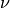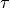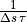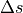# Velocity Verlet with Boltzmann distribution¶

This integrator combines the velocity-Verlet algorithm and the Boltzmann velocity distribution. At a periodic interval, the velocities are assigned from a Boltzmann velocity distribution in the second integration half-step.

This integrator is especially useful for cooling or heating a system to a nominal temperature. After an assignment from the Boltzmann distribution, the centre of mass velocity is shifted to exactly zero, and the velocities are rescaled to exactly the nominal temperature.

class halmd.mdsim.integrators.verlet_nvt_boltzmann(args)

Construct integrator for given system of particles.

Parameters: args (table) – keyword arguments args.particle – instance of halmd.mdsim.particle args.box – instance of halmd.mdsim.box args.timestep (number) – integration time step (defaults to halmd.mdsim.clock.timestep) args.temperature (number) – temperature of Boltzmann distribution args.rate (number) – nominal coupling rate
set_timestep(timestep)

Set integration time step in MD units.

Parameters: timestep (number) – integration timestep

This method forwards to halmd.mdsim.clock.set_timestep(), to ensure that all integrators use an identical time step.

timestep

Integration time step in MD units.

temperature

Temperature of Boltzmann distribution in MD units.

interval

Coupling interval in steps.

The interval equals, with nominal coupling rateand time-step.

rate

Effective coupling rate per time in MD units.

The effective coupling rate equals, with coupling intervaland time-step.

set_temperature(temperature)

Set the temperature of the Boltzmann distribution to the given value.

integrate()

Calculate first half-step.

By default this function is connected to halmd.mdsim.core.on_integrate().

finalize()

Calculate second half-step, or assign velocities from Boltzmann distribution.

By default this function is connected to halmd.mdsim.core.on_finalize().

disconnect()

Disconnect integrator from core and profiler.